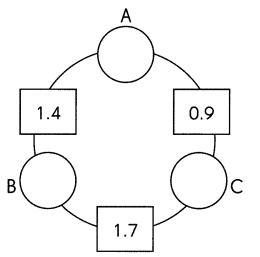Practice the problems of Math in Focus Grade 4 Workbook Answer Key Chapter 8 Adding and Subtracting Decimals to score better marks in the exam.

Challenging Practice

Question 1.
Miguel subtracts two numbers and gets the answer 4.95. The lesser of the two numbers is 3.4. What is the other number?
The other number = 8.35.

Explanation:
Miguel subtracts two numbers and gets the answer 4.95.
Let the two number s be X and Y.
=> X – Y = 4.95.
The lesser of the two numbers is 3.4.
=> X – 3.4 = 4.95
=> X = 4.95 + 3.4
=> X = 8.35.

Question 2.
Julia subtracts two numbers and gets the answer 6.8. The greater of the two numbers is 10.55. What is the other number?
The other number = 3.75.

Explanation:
Julia subtracts two numbers and gets the answer 6.8.
Let the two number s be X and Y.
=> X – Y = 6.8.
The greater of the two numbers is 10.55.
=> 10.55 – Y = 6.8
=> 10.55 = 6.8 + Y
=> 10.55 – 6.8 = Y
=> 3.75 = Y

Problem Solving
Question 1.
The number in each rectangle is the sum of the numbers in the two circles next to it. Find the numbers in the circles.A = 0.3.
B = 1.1
C = 0.6

Explanation:
The number in each rectangle is the sum of the numbers in the two circles next to it.
=> Equation 1: A + B = 1.4
=> Equation 2: A + C = 0.9
=> Equation 3: B + C = 1.7.
Equation 1 – Equation 2
=> (A + B) – (A + C ) = 1.4 – 0.9
=> A + B – A – C = 0.5
=> B – C = 0.5.
=> B = 0.5 + C.
Equation 3 – Equation 2:
(B + C) – (A + C ) =  1.7 – 0.9
=> B + C – A – C = 0.8
=> B – A = 0.8.
=> A = B – 0.8.
Substitute A and B in Equation:1.
=> A + B = 1.4
=> B – 0.8 + B = 1.4
=> 2B – 0.8 = 1.4
=> 2B = 1.4 + 0.8
=> 2B = 2.2
=> B = 2.2 ÷ 2
=> B = 1.1.
A = 1.1 – 0.8
=> A = 0.3.
Substitute A = 0.3 in Equation 2:
=> A + C = 0.9
=> 0.3 + C = 0.9
=> C = 0.9 – 0.3
=> C = 0.6.

Question 2.
Each week, Rena saves $5. Her brother saves$2.50 less each week, but he started saving 4 weeks earlier. After how many weeks will Rena’s savings be equal to her brother’s?
Amount of money Rena saves each week = $5. Her brother saves$2.50 less each week, but he started saving 4 weeks earlier.
Amount of money her brother saves each week = Amount of money Rena saves each week – $2.50. =$5 – $2.50 =$2.50.
= 4 × $2.50 =$10.
= $10 ÷$5